# The essence of Monte Carlo

Monte Carlo methods (MC) are a series of mathematical methods through which we can gain insight into the statistical properties of a system by running repeated independent trials and stacking them up against each other. These methods find plenty of uses in areas like engineering, physics, finance, data science, machine learning, and more. However, MC methods can be very challenging to understand, and lack of understanding often leads to the wrong implementation, which ultimately leads to either erroneous results or erroneous interpretations of those results. In this article, I present a very brief introduction to the essence of MC methods, and how they can be used to estimate physical quantities based on randomised trials with nothing other than a computer, and a little bit of Matlab programming.

As a simple example, I will approximate the value of Pi (e.g. 3.14159265359…) by throwing darts unto a square board of area 2 x 2. In it, there is an inscribed circle of radius 1, which fits exactly into the square. The basic idea is this: we throw in a certain number N of darts randomly into the square, and count how many land inside the circle, as shown in Figure 1.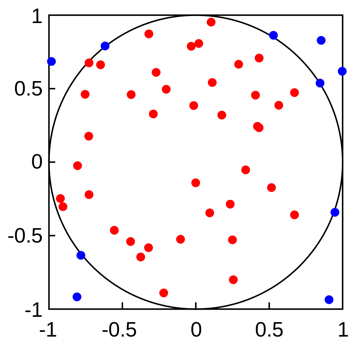Figure 1: random darts thrown at a circle inside a square

We will mark the “hits” (those darts whose center lands inside the circle) using red dots, and the “misses” using blue dots. To estimate the value of Pi from this, we simply note that the area of a circle is Area = Pi x Radius², from which we simply write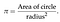Equation 1: calculating Pi based on the area of a circle and its radius

In our case, the area of the circle is estimated from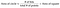Equation 2: estimating the area of a circle using random points

We expect that as we increase the number of darts N we should get a better estimate of Pi each time. However, it is not sufficient to just do this “virtual experiment” once. In fact, the essence of Monte Carlo is to repeat this experiment many times, say M times, such that we get an average value of Pi. This essentially means that our estimate’s accuracy will depend on both our choice of N and M.

As an example of how we would achieve this programmatically, here is a snippet of Matlab code I made: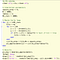Figure 1: Matlab code for estimating the value of Pi using Monte Carlo simulations

The idea here is simple: at the beginning of each Monte Carlo loop, denoted by the index jj, I am initializing the value of ‘hits’ to zero. Then, inside the next loop where I throw N darts on the board, I create a set of uniformly distributed random numbers on the domain [-1,1] for both the x and y coordinates. I then use an if-statement to test whether these coordinates (x,y) fall within the circle of radius 1. I then calculate the Pi estimate for each Monte Carlo run based on the number of hits recorded, and store this away into the array “pi_approximate”. Once all the Monte Carlo runs are done, I calculate “pi_avg” based on the mean value of all the Pi estimates I stored. For a single run of this code, I get the following output: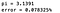Figure 2: Output from the Matlab code in Figure 1

As you can see, the error % is very small, but this only means that our estimate for Pi is accurate to within 2 decimal places, which seems less than ideal. Getting higher accuracy to more decimal places would require us to increase both N and M quite a lot, which would put a lot of strain on our computer.

Monte Carlo methods are quite useful in a lot of applications, but one must be careful in how the simulations are set up because accuracy can be quite a problem depending on what we are trying to calculate. In practice, Monte Carlo calculations are best performed in parallel, using either multi-core CPUs or GPUs (GPUs tend to have a lot more independent workers than CPUs, but each one has less computational power, so there is usually a tradeoff between speed and efficiency)

Oscar is a physicist, educator and STEM enthusiast. He is currently finishing a PhD in Theoretical Physics with a focus on photonics and stochastic dynamics.

## More from Oscar Nieves

Oscar is a physicist, educator and STEM enthusiast. He is currently finishing a PhD in Theoretical Physics with a focus on photonics and stochastic dynamics.